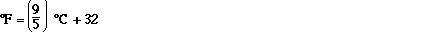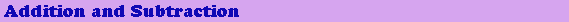### The most useful approach to these types of problems is to use dimensional analysis or unit analysis where equivalences are expressed as ratios and used to convert from one unit to another. Given the two equivalence above we can write two ratios,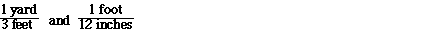### The unit conversion factor for this definition is;### Either can be used depending on the direction of conversion. Given the number of centimeters the first unit conversion factor can be used to convert centimeters to meters. Given the number of meters the second unit conversion factor can be used to convert meters to centimeters. For example;### The unit conversions in this case are exact numbers. We do not have to worry about the number of significant figures in the unit conversion. However, there are conversion factors which are not exact and we must use more care. The conversion of yards to meters is an example. We know that### The number of significant figures in the given quantity will determine the correct number of significant figures in the unit conversion. You may wish to use all of significant figures in the calculation and than round the answer to the correct number of significant figures. There is a useful list of important relationships which can be used a conversion factors inside the back cover of your textbook.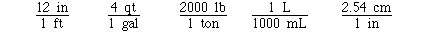### Some other exact unit conversions include;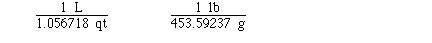### Quantities expressed in compound units are a little more interesting. Density is a good examples of a compound unit. For example the density of gold is;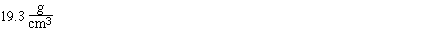###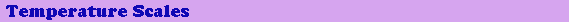### There is a relationship between the Fahrenheit scale and the Celsius scale which is given as,